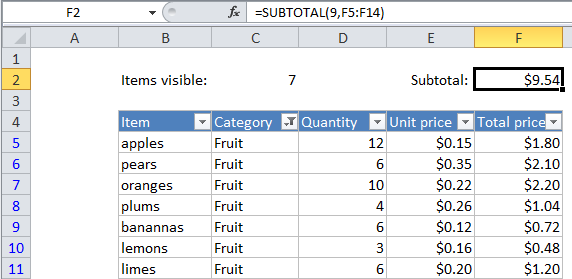## Excel Office

Excel How Tos, Tutorials, Tips & Tricks, Shortcuts

# Sum visible rows in a filtered list in Excel

This tutorial shows how to Sum visible rows in a filtered list in Excel using the example below;

## Formula

`=SUBTOTAL(9,range)`## Explanation

If you want to sum only the visible rows in a filtered list (i.e. only those rows not filtered out), you can use the SUBTOTAL function with function number 9 or 109. What makes SUBTOTAL especially useful is that it automatically ignores rows that are hidden in a filtered list or table.

Also See:   Count visible rows only with criteria in Excel

Following the example in the screen above, to sum cells in column F for visible rows only, use:

`=SUBTOTAL(9,F5:F14)`

If you are hiding rows manually (i.e. right-click, Hide), use this version instead:

`=SUBTOTAL(109,F5:F14)`

By changing the function number, the SUBTOTAL function can perform many other calculations (e.g. COUNT, SUM, MAX, MIN, etc.).

Also See:   Count visible rows in a filtered list in Excel